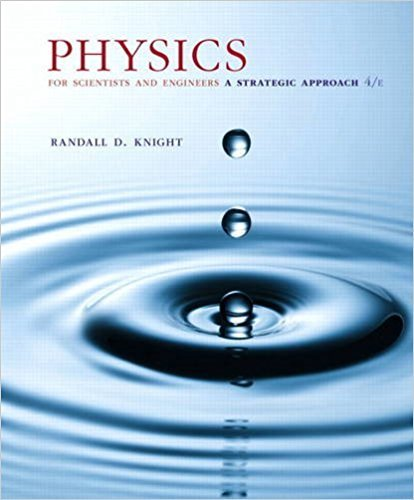×
×

# Newtons law of gravity and Coulombs law are bothISBN: 9780134081496 191

## Solution for problem 24.83 Chapter 24

Physics for Scientists and Engineers: A Strategic Approach, Standard Edition (Chs 1-36) | 4th Edition

• Textbook Solutions
• 2901 Step-by-step solutions solved by professors and subject experts
• Get 24/7 help from StudySoup virtual teaching assistantsPhysics for Scientists and Engineers: A Strategic Approach, Standard Edition (Chs 1-36) | 4th Edition

4 5 0 299 Reviews
28
3
Problem 24.83

Newtons law of gravity and Coulombs law are both inversesquare laws. Consequently, there should be a Gausss law for gravity. a. The electric field was defined as E u = F u on q /q, and we used this to find the electric field of a point charge. Using analogous reasoning, what is the gravitational field g u of a point mass?Write your answer using the unit vector nr, but be careful with signs; the gravitational force between two like masses is attractive, not repulsive. b. What is Gausss law for gravity, the gravitational equivalent of Equation 24.18? Use G for the gravitational flux, g u for the gravitational field, and Min for the enclosed mass. c. A spherical planet is discovered with mass M, radius R, and a mass density that varies with radius as r = r011 - r/2R2, where r0 is the density at the center. Determine r0 in terms of M and R. Hint: Divide the planet into infinitesimal shells of thickness dr, then sum (i.e., integrate) their masses. d. Find an expression for the gravitational field strength inside the planet at distance r 6 R.

Step-by-Step Solution:
Step 1 of 3

Weekly Assignment 09 https://session.masteringphysics.com/myct/assignmentPrintViewdispl... Weekly Assignment 09 Due: 9:00pm on Friday, March 25, 2016 To understand how points are awarded, read theading Policy for this assignment. Focusing with the Human Eye Joe is hiking through the woods when he decides to stop and take in the...

Step 2 of 3

Step 3 of 3

##### ISBN: 9780134081496

The full step-by-step solution to problem: 24.83 from chapter: 24 was answered by , our top Physics solution expert on 12/28/17, 08:06PM. Physics for Scientists and Engineers: A Strategic Approach, Standard Edition (Chs 1-36) was written by and is associated to the ISBN: 9780134081496. Since the solution to 24.83 from 24 chapter was answered, more than 577 students have viewed the full step-by-step answer. This textbook survival guide was created for the textbook: Physics for Scientists and Engineers: A Strategic Approach, Standard Edition (Chs 1-36), edition: 4. The answer to “Newtons law of gravity and Coulombs law are both inversesquare laws. Consequently, there should be a Gausss law for gravity. a. The electric field was defined as E u = F u on q /q, and we used this to find the electric field of a point charge. Using analogous reasoning, what is the gravitational field g u of a point mass?Write your answer using the unit vector nr, but be careful with signs; the gravitational force between two like masses is attractive, not repulsive. b. What is Gausss law for gravity, the gravitational equivalent of Equation 24.18? Use G for the gravitational flux, g u for the gravitational field, and Min for the enclosed mass. c. A spherical planet is discovered with mass M, radius R, and a mass density that varies with radius as r = r011 - r/2R2, where r0 is the density at the center. Determine r0 in terms of M and R. Hint: Divide the planet into infinitesimal shells of thickness dr, then sum (i.e., integrate) their masses. d. Find an expression for the gravitational field strength inside the planet at distance r 6 R.” is broken down into a number of easy to follow steps, and 190 words. This full solution covers the following key subjects: . This expansive textbook survival guide covers 42 chapters, and 4463 solutions.

Unlock Textbook Solution